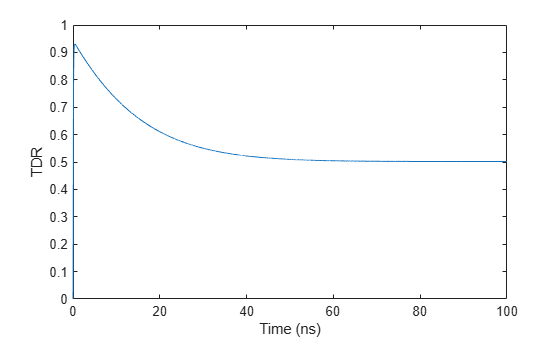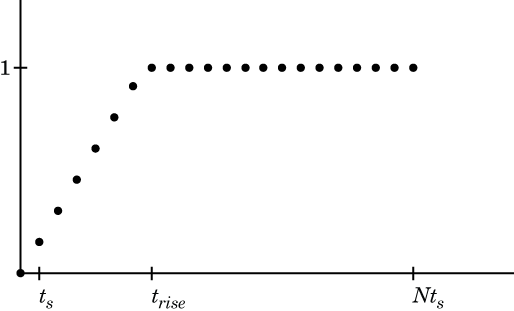Main Content

# stepresp

Step-signal response for rational object and `rationalfit` function object

## Syntax

``[outputsignal,tout] = stepresp(h,ts,n,trise)``

## Description

example

````[outputsignal,tout] = stepresp(h,ts,n,trise)` computes the time domain response of a rational function object, `h`, to a step signal based on the number of samples, `n` and the rise time, `trise`.```

## Examples

collapse all

Calculate the step response of a rational function object from the file `passive.s2p`. Read `passive.s2p`.

```S = sparameters('passive.s2p'); freq = S.Frequencies;```

Get S11 and convert to a TDR transfer function.

```s11 = rfparam(S,1,1); Vin = 1; tdrfreqdata = Vin*(s11+1)/2;```

Fit to a rational function object.

`tdrfit = rationalfit(freq,tdrfreqdata);`

Define parameters for a step signal. Define parameters for a step signal

```Ts = 1.0e-11; N = 10000; Trise = 1.0e-10;```

Calculate the step response for TDR and plot it

```[tdr,t1] = stepresp(tdrfit,Ts,N,Trise); figure plot(t1*1e9,tdr) ylabel('TDR') xlabel('Time (ns)')```## Input Arguments

collapse all

Rational function object, specified as a `rationalfit` object handle.

Example:

Data Types: `double`
Complex Number Support: Yes

Sample time of the input signal, specified as a positive scalar integer in seconds.

Example:

Data Types: `double`

Number of samples, specified as a positive scalar integer.

Example:

Data Types: `double`

Time taken for step signal to reach maximum value, specified as a positive scalar integer in seconds.

Example:

Data Types: `double`

## Output Arguments

collapse all

Output signal,

Example:

Data Types: `double`
Complex Number Support: Yes

Sample time of the output signal, returned as a positive scalar integer in seconds.

Example:

Data Types: `double`

## More About

collapse all

### Step Signal Equation

RF Toolbox™ uses the following equation to for the step signal:

`$\left\{\begin{array}{l}U\left(k{t}_{s}\right)=k{t}_{s}/{t}_{rise},\\ U\left(k{t}_{s}\right)=1,\end{array}\text{ }\text{ }\begin{array}{c}0\le k<\left({t}_{rise}/{t}_{s}\right)\\ \left({t}_{rise}/{t}_{s}\right)\le k\le N\end{array}$`

The following figure illustrates the construction of this signal.## See Also

Introduced in R2010a

Download ebook